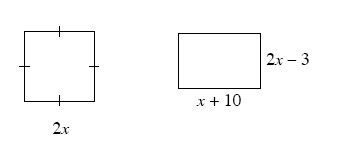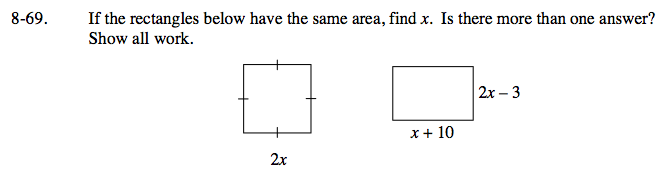Home > GC > Chapter 8 > Lesson 8.2.1 > Problem8-69

8-69.

If the rectangles below have the same area, find x. Is there more than one answer? Show all work. Homework Help ✎If the areas are the same, how can you set up an equation to solve for x?

Based on this equation, can there be two answers?
Remember with the area of a rectangle being base × height, you end up with (2x)(2x) = (x + 10)(2x − 3).